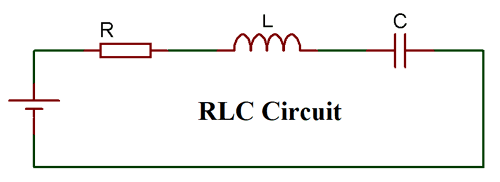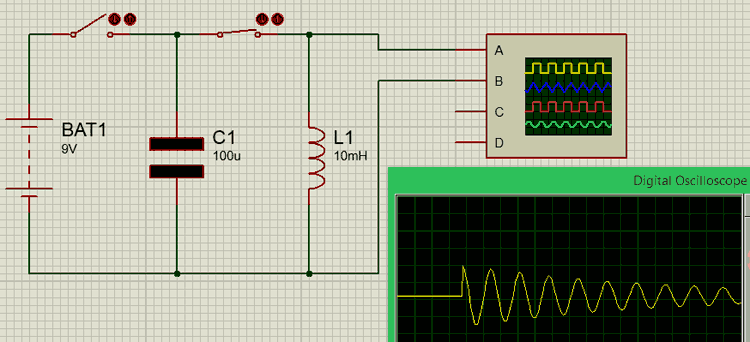# RLC Circuit Calculator

### OUTPUT

 Frequency HertzKiloHertzMegaHertz Q Factor

With the RLC circuit calculator, you can calculate the resonant frequency and the Q-factor of any RLC circuit by providing capacitance, inductance and resistance values.

### RLC circuit

RLC circuit as the name implies consist of a Resistor, Capacitor and Inductor connected in series or parallel. The circuit forms an Oscillator circuit which is very commonly used in Radio receivers and televisions. It is also very commonly used as damper circuits in analog applications.The RLC circuit is also called as series resonance circuit, oscillating circuit or a tuned circuit. These circuit has the ability to provide a resonant frequency signal as shown in the below image:Formula for the resonant frequency of the RLC circuit:

Below is the formula to calculate the resonant frequency of a RLC circuit:

`f = 1 / [2π * √(L * C)]`

where:

• f is the resonant frequency.
• L is the impedance of the inductor.
• C is the capacitance of the capacitor.

Calculating Q Factor of the RLC circuit:

The Q factor or quality factor shows the quality of the RLC circuit. While designing a RLC circuit, one should aim to achieve the higher Q-factor. Below is the formula for the Q-factor of a RLC circuit:

`Q = 1/R * √(L/C)`

where:

• Q is the Q-factor
• R is the resistance.